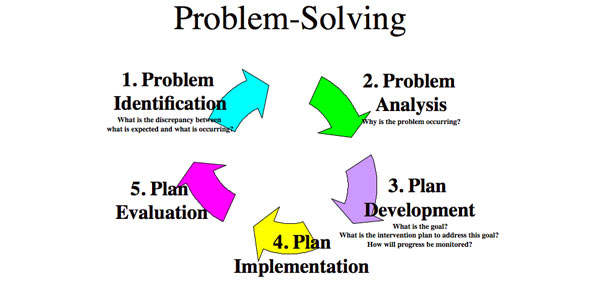# Period 5 Horizontal Projectile Practice

7 Questions | Total Attempts: 41SettingsWalks you through solving a horizontal projectile problem.

• 1.
A dog chases a ball off of a low cliff, running 13 m/s. She splashes into the water, which is 11 m below. How far away from the base of the cliff did the dog splash into the water? Which variables belong on the x side of the T chart? Check all that apply.
• A.

V= 13 m/s

• B.

Vi=0 m/s

• C.

A= -9.8 m/s

• D.

D= 11m

• E.

D= -11 m

• F.

Vf=0 m/s

• 2.
A dog chases a ball off of a low cliff, running 13 m/s. She splashes into the water, which is 11 m below. How far away from the base of the cliff did the dog splash into the water? Which variables belong on the y side of the T chart? Check all that apply.
• A.

V= 13 m/s

• B.

Vi=0 m/s

• C.

A= -9.8 m/s/s

• D.

D= 11m

• E.

D= -11 m

• F.

Vf=0 m/s

• 3.
A dog chases a ball off of a low cliff, running 13 m/s. She splashes into the water, which is 11 m below. How far away from the base of the cliff did the dog splash into the water? Which equation will you be using on the x side of the T chart?
• A.

Constant acceleration equation 1

• B.

V=d/t

• C.

Constant acceleration equation 2

• D.

Constant acceleration equation 3

• E.

Constant acceleration equation 4

• 4.
A dog chases a ball off of a low cliff, running 13 m/s. She splashes into the water, which is 11 m below. How far away from the base of the cliff did the dog splash into the water? Which variable should you try to solve for first?
• A.

Vf

• B.

Vi

• C.

D d

• D.

T

• E.

A

• 5.
A dog chases a ball off of a low cliff, running 13 m/s. She splashes into the water, which is 11 m below. How far away from the base of the cliff did the dog splash into the water? Which equation will you be using on the y side of the T chart?
• A.

V=d/t

• B.

Constant acceleration equation 1

• C.

Constant acceleration equation 2

• D.

Constant acceleration equation 3

• E.

Constant acceleration equation 4

• 6.
A dog chases a ball off of a low cliff, running 13 m/s. She splashes into the water, which is 11 m below. How far away from the base of the cliff did the dog splash into the water? How long will it take the dog to hit the water?
• A.

1.05 s

• B.

1.49 s

• C.

2.2 s

• D.

This problem cannot be solved

• E.

Constant acceleration equation 4

• 7.
A dog chases a ball off of a low cliff, running 13 m/s. She splashes into the water, which is 11 m below. How far away from the base of the cliff did the dog splash into the water? How far will the dog land from the bottom of the cliff
• A.

8.72 m

• B.

0.11 m

• C.

19.37 m

• D.

This problem cannot be solved

Related TopicsBack to top